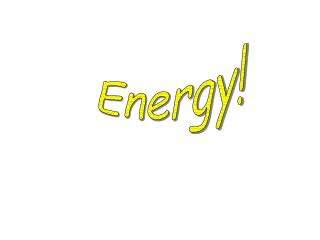DownloadDownload PresentationEnergy!

# Energy!

Télécharger la présentation## Energy!

- - - - - - - - - - - - - - - - - - - - - - - - - - - E N D - - - - - - - - - - - - - - - - - - - - - - - - - - -
##### Presentation Transcript

1. Energy!

2. Brainstorm Energy ENERGY

3. 7 Forms of Energy • Heat • Chemical • Light • Electrical • Mechanical • Sound • Nuclear

4. Energy • The ability to do work • Involves either motion or position • There are many different types of energy • Law of Conservation of Energy states that Energy cannot be created nor destroyed only transformed from one form to another!

5. In your notes… Energy = the ability to do work Renewable Resources = Can get back Nonrenewable Resources = Cannot get back Conservation = conserving/saving our resources

6. Energy Resources • Renewable and nonrenewable forms of energy • Fossil Fuels (Nonrenewable) • Solar (Renewable) • Wind (Renewable) • Water (Renewable) • Nuclear (Renewable) • Geothermal (Renewable) • Biomass (Renewable)

7. Renewable Resources • Natural resources that CAN be replaced by natural processes in a fairly short amount of time • Examples: Wind, Sun, Biomass, Nuclear, Water, Geothermal, etc.

8. Nonrenewable Resources • Natural resources that either CANNOT be replaced or which may take millions of years to be replaced by natural processes. • Examples: Fossil Fuels - Oil, Coal, Gas, and Some Minerals

9. Create 7 of these in your Notes- One for each type of Energy! Definition: Examples: Picture or Symbol: Interesting Info:

10. Heat Energy Definition: the energy of moving particles of matter Examples: Solar, Fire, Chemical, Body, Electrical, Friction, Nuclear, Geothermal,etc Pic/Symbol: Interesting Info: There is more Heat Energy in an Iceberg than there is in a boiling pot of water!

11. Heat vs. Temperature Heat= the number of molecules Temperature= the motion of molecules Which has more Heat? Which has more Temp? Boiling pot of water vs. Iceberg Ice Sculpture vs. Lit Match

12. Chemical Energy Definition: Energy stored in the chemical compounds of food & fuel Examples: Fossil Fuels (Oil, Gas, & Coal) Wood, Stored in food, etc. Pic/Symbol: Info: One of our most abundant and important sources of energy!

13. Light Energy Definition: Energy from the Electromagnetic Spectrum (EMS) Examples: Sun, light bulb, lightning, etc. Picture/Symbol: Interesting Information: Light is both a particle (photon) and a wave!

14. Electrical Energy Definition: The energy of moving electrons. Examples: Power plant, batteries, static, current, etc. Picture/Symbol: Interesting Information: Alternating Current= when you plug something in! Direct Current= Battery (1 direction – to +)

15. Mechanical Energy Definition:The energy of an object that is due to its position (potential) or motion (kinetic). Examples: Roller coaster at top of hill PE & Roller coaster going down hill KE Picture/Symbol: Interesting Information:Mechanical Energy allows an object to do work! Mechanical NRG = Potential NRG + Kinetic NRG

16. Sound Energy Definition: Energy created by vibrating objects. Examples: sound from a speaker, radio, TV, wind, thunder, or someone’s voice Picture/Symbol: Interesting Information: Sound travels through a medium and the speed of sound depends on the density of the medium!

17. Nuclear Energy • Definition: Uses atomic reactions to produce electricity. Examples: • Fission (split) and fusion (fuse) of radioactive nuclei Picture/Symbol: Interesting Information: E = mc2 • This mass can be changed into energy under the proper conditions according to Albert Einstein's famous equation: where E = energy, m=mass, and c=speed

18. Potential Energy • Potential energy is stored energy/energy of position. • Ex) • The Potential Energy Equation • PE = mass x gravity x height • m = mass (in kg), g = gravity (9.8m/s2), and height (in meters) = potential energy (in J = joules). • There is an inverse relationship between potential and kinetic energy • As PE increase KE decreases and as PE decreases KE increases.

19. Kinetic Energy • Kinetic energy is the energy of motion. • Any object that is moving has kinetic energy. • Ex) Ball Moving, Wind, Sound • The Kinetic Energy Equation • KE= ½ mv2 • m = mass (in kg), v = velocity (in m/s), and KE = kinetic energy (in J = joules). • There is a direct relationship between KE and mass and between KE and v squared. • Therefore as the mass goes up, KE goes up. And as v goes up, KE goes up.